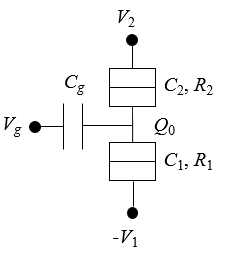## I-Vg characteristics of a single-electron transistor

 The current through a single electron transistor is a periodic function of the gate voltage. The transistor consists of a conducting island coupled to two tunnel junctions and a gate capacitor. The tunnel junctions have capacitances $C_1$, $C_2$ and resistances $R_1$, $R_2$. The gate capacitance is $C_g$ and the offset charge on the island is $Q_0$. Bias voltages of $V_1$ and $V_2$ are applied to the tunnel junctions. The form below can be used to plot the $I-V_g$ characteristics at temperature $T$ from $V_g$(start) to $V_g$ (stop). $N$ is the number of points used in the plot. The equations that are used are described in Günther Lientschnig, Irek Weymann and Peter Hadley, Simulating Hybrid Circuits of Single-Electron Transistors and Field-EffectTransistors, Japanese Journal of Applied Physics 42 pp. 6467-6472 (2003).$I$ [nA] $V_g$ [mV]
 $C_1$ = F $C_2$ = F $R_1$ = $\Omega$ $R_2$ = $\Omega$ $C_{g}$ = F $V_{g}$ (start) = V $V_{g}$ (stop) = V $Q_0$ = $e$ $T$ = K $V_1$ = V $V_2$ = V $N$ =

 $V_g$ [V] $I$ [A]